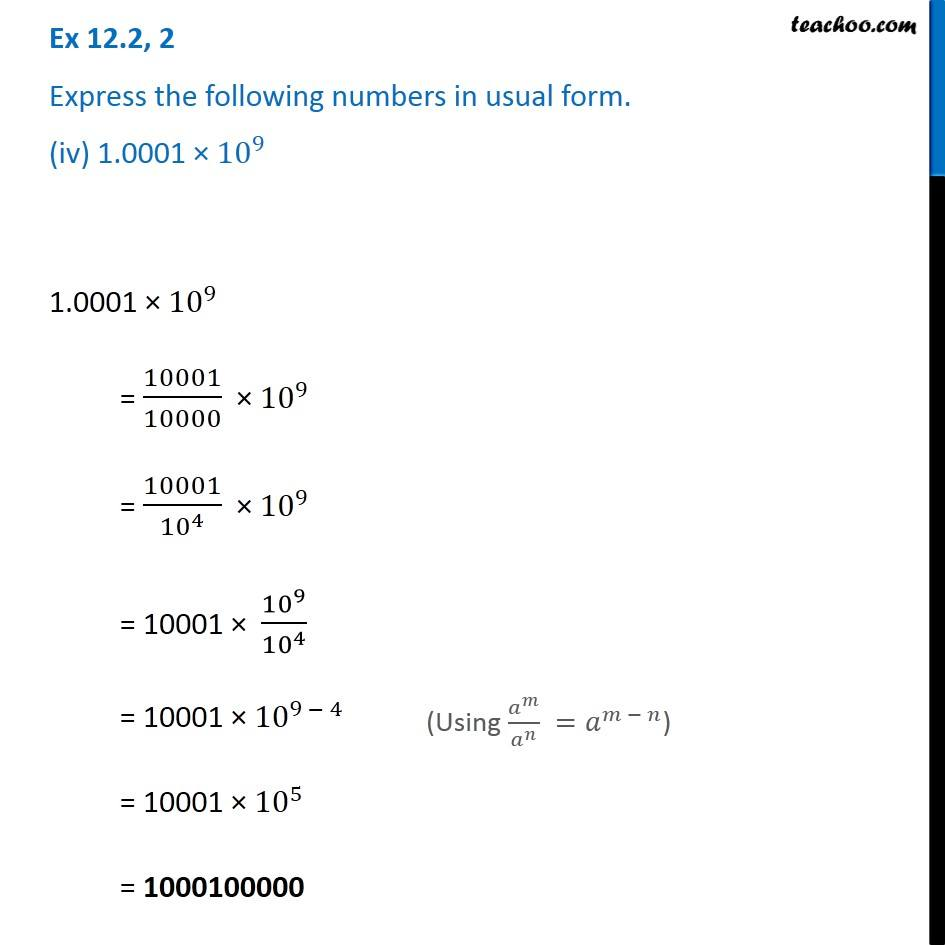Ex 12.2

Chapter 12 Class 8 Exponents and Powers
Serial order wiseIntroducing your new favourite teacher - Teachoo Black, at only ₹83 per month

### Transcript

Ex 12.2, 2 Express the following numbers in usual form. (iv) 1.0001 × 〖10〗^9 1.0001 × 〖10〗^9 = 10001/10000 × 〖10〗^9 = 10001/〖10〗^4 × 〖10〗^9 = 10001 × 〖10〗^9/〖10〗^4 = 10001 × 〖10〗^(9 − 4) = 10001 × 〖10〗^5 = 1000100000 (Using 𝑎^𝑚/𝑎^𝑛 =𝑎^(𝑚 − 𝑛))